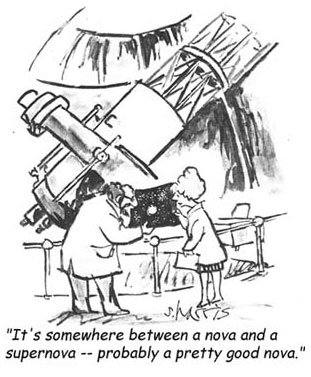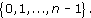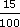The data in Table 3 are the recorded birth weights of 50 infants who displayed severe idiopathic respiratory distress syndrome (SIRDS). This is a serious condition which can result in death.

## Author(s): The Open UniversityLicense informationRelated contentCopyright Â© 2016 The Open University

When calculating an answer it is important that you give careful consideration to the order of operations used in the calculation. If you are using a mixture of operations remember that certain operations take priority in a calculation. Consider the following, apparently, simple sum.

1 + 2 × 3 = ?

What answer would you give?

Did you give 7 as your response, or 9?

The correct answer is 7 but can you explain why?

If you have a calculator handy, check that it
Author(s): The Open University

## Activity 14

Measurement of a ceiling gives a length of 6.28 m and a width of 3.91 m.

• (a) Make a rough estimate of the area of the ceiling (the length times the width).

Author(s): The Open UniversityApproximations are most useful when it comes to making rough estimates –
Author(s): The Open University

Sometimes it doesn’t make sense to round to a specific number of decimal places. If, say, you were calculating the cost of fencing at £10.65 per metre, for a garden boundary, the length of which had been given to you as 185 feet, then you would want to multiply 10.65 × 185 × 0.3048. (Conversion of feet to metres was given in Author(s): The Open University

• 1.   The condition ‘is equal to’ is a relation on any set of real numbers because, for any x, y in the set, the statement ‘x is equal to y’ is either definitely true or definitely false. This relation is usually denoted by the symbol =. For this relation, each real number in the set is related only to itself!

• 2.   The condition ‘is less than’ is a relation on any set of real numbers, and we usually
Author(s): The Open University

The Division Algorithm tells us that all the possible remainders on division by an integer n lie in the setWe denote this set by [Image_Link]https://www.open.edu
Author(s):
The Open University

We will now discuss complex numbers and their properties. We will show how they can be represented as points in the plane and state the Fundamental Theorem of Algebra: that any polynomial equation with complex coefficients has a solution which is a complex number. We will also define the function exp of a complex variable.

Earlier we mentioned several sets of numbers, including [Image_Link]https://www.open.edu/openlearn/ocw/pluginfile.php/89651/mod_ouc
Author(s):
The Open University## Study another free course

There are more than 800 courses on OpenLearn for you to
Author(s): The Open University

An important idea when calculating volumes of simple shapes is that of a cross-section. In the case of the rectangular box considered above, it is possible to slice through the box horizontally so that the sliced area is exactly the same as the area of the base or top; in other words, the areas of the horizontal cross-sections are equal.

[Image_Link]https://www.open.edu/openlearn/ocw/pluginfile.php/94675/mod_oucontent/ouco
Author(s): The Open University

What is a volume? The word usually refers to the amount of three-dimensional space that an object occupies. It is commonly measured in cubic centimetres (cm3) or cubic metres (m3).

A closely related idea is capacity; this is used to specify the volume of liquid or gas that a container can actually hold. You might refer to the volume of a brick and the capacity of a jug – but not vice versa. Note that a container with a particular volume will not nec
Author(s): The Open University

## Question 1

Find the area of a circle of (a) radius 8 cm, and (b) radius 15 m.

### Answer

• (a)

Author(s): The Open University

## Question 1

Find the area of each of these shapes.

[Image_Link]https://www.open.edu/openlearn/ocw/pluginfile.php/94675/mod_oucontent/oucontent/779/39f826a0
Author(s): The Open University

In this section we shall define the complex number system as the set R × R (the Cartesian product of the set of reals, R, with itself) with suitable addition and multiplication operations. We shall define the real and imaginary parts of a complex number and compare the properties of the complex number system with those of the real number system, particularly from the point of view of analysis.

Author(s): The Open University

Section 6 contains solutions to the exercises that appear throughout sections 1-5.

Click the link below to open the solutions (13 pages, 232KB).

Section 6

Author(s): The Open University

In Section 5 we show how functions may be used to sketch curves in the plane, even when these curves are not necessarily the graphs of functions.

Click the link below to open Section 5 (8 pages, 151KB).

Section 5

Author(s): The Open University

Discount can be calculated in the same way as an increase by a percentage. For example, £8 with 15% discount means you actually pay

£8 less (15% of £8)

15% of 8 =× 8 =
Author(s): The Open University

Fractions and decimals can also be converted to percentages, by multiplying by 100%.

So, for example, 0.17, 0.3 andcan be expressed as percentages as follows:

0.17 × 100% = 17%;

<
Author(s): The Open University

## Activity 3

A local supermarket sells a popular breakfast cereal in a ‘Large Pack’ and ‘New Extra Large Pack’. They are both being sold at ‘knock down’ prices. The large pack contains 450 g of cereal priced at �
Author(s): The Open University